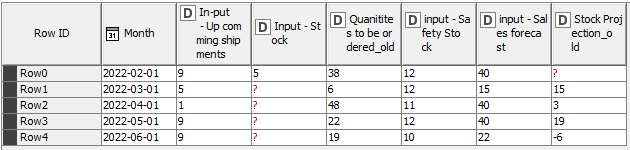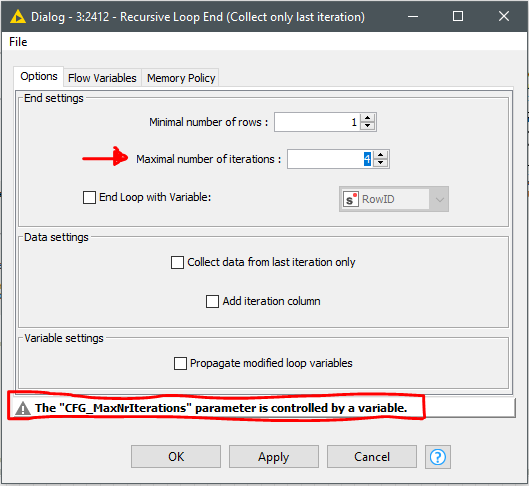# How to create a loop between to equations using one's input as the other's output

Hello, I am working with a system of two equations where one’s input will be used as the other’s output. The first equation calculates the quantities to order for a month (n), and the second one the stock projection for the next month (n+1). This later will be injected as an input to calculate the ordering quantities for the month n+1 which in turn will be injected into the second equation once again to calculate the stock projections for n+2 which again will be input to calculate the stock projection for month n+3… and this loop should be repeated over the span of 12 month.

Following are the two equation:
Quatities to be ordered (n): safety stock (n+1) + sales projections (n) - up comming
Nouveau Feuille de calcul Microsoft Excel.xlsx (14.7 KB)
shipments (n) - stock (n)
Stock projections (n+1): stock (n) + up comming shipments (n) + quantites to be ordered (n) - sales projection (n).

The known variables are:
Safety sotck (all months), sales forecast (all months), up comming shipments (all months), stock (only first month).

I guess the best option here is to use a recursive loop where the output results from the 1st equation are reused as new data for the second equation during the next recursive iteration.

Thus,

• Odd recursive iterations should execute the 1st equation with the generated results from 2nd equation, whilst
• Even recursive iterations should execute the 2nd equation with 1st equation generated results as input data.
until the expected results are obtained or an ending condition is reached.

Hope it helps to devise a convenient algorithm.

Best

Ael

2 Likes

Hello @aworker Thank you for your suggestion. The thing is that I need to get the output (stock projection and quantity to be ordered) for each month in the process, not only the projection and stock for the last month in the loop

@mehdi_zinbi
@aworker proposed recursion loop allows collecting the data from each recursion and stack them in the output (if that helps)
br

1 Like

Hello @mehdi_zinbi
Any progress with this challenge?

I’ve been having a look to provided data and to the challenge description as well. And I have a couple of comments related to it:

1. The caption of the challenge is referred to the conceptual calculation method, and asking about an approach to achieve the expected results. However the data gather and transformation just to get something like a structured starting object, it’s a topic by itself and requires some added efforts.
Then, if you expect a quick and effective help from others in the Forum you should provide the challenge data in shape for the proposed challenge.

2. Regarding the challenge itself, the easiest would be to code it (R?). However I think that a possible approach by using just KNIME nodes is doable; it requires to address some other issues commented in many posts before, like column name handling inside a loop…
From my perspective, the approach is a nested recursive loop handling the ‘Stock projections’ calculation within an standard loop operating over the items (Ships, Banana, Apples…)

BR

1 Like

Hello @mehdi_zinbi
I’ve finally started to put together some nodes and drew down a workflow, anticipated in my previous post. Part of the workflow solved all the data-mining issues, when gathering the data in shape for the analysis ambition. But we can just concentrate on the proposed solution for your calculation constrains.

This picture shows the recursive loop that operates the two equation. The column names are normalized on every main Loop step, then the equations will always operate with standardized column names within the Nested Recursive Loop . I’ve numbered in red the following comments.

.

1. Lets focus on the first Iteration (Item == Ships). The data is formatted to wide in columns with time series ordered in a reference column. See input data.2. On the first step of the loop the equations and support nodes calculate the required parameters [ f(1) ‘Quantities to be ordered’ ] and [ f(2) ‘Stock Projections’ ] for the first row in time line. And typing the ‘Stock Projection (n+1)’ calculated value to the second row ‘Input Stock’ column. Then only first row is saved to loop end gather and updating the rank (\$rank\$ - 1) for the next loop step.

3. As one of the inputs is ‘Input - Safety Stock (n+1)’ and it isn’t available for the last row (June month in this case); the loop is programmed to run just for 4 iterations (this is Max. \$rank\$ - 1).4. Then the final output just extends until the 4th iteration (this is the May month). This matrix shows the final output for the first item (Ships), collected on a row by row iteration.

I hope that this workflow can be extrapolated to your real data

BR

PS.- Sorry @ScottF for deleting the previous post, but I spotted an error in the workflow and I needed few minutes to fix it.

2 Likes

Thank you for your help, I really appreciate it. I will give it a try and come back to you.

This topic was automatically closed 90 days after the last reply. New replies are no longer allowed.